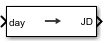Documentation

# Julian Date Conversion

Calculate Julian date or modified Julian date

## Library

Utilities/Unit Conversions## Description

This block converts the specified date to the Julian date or modified Julian date.

## Parameters

Year

Specify the year used to calculate the Julian date. Enter a double value that is a whole number greater than 1, such as `2013`.

Month

Specify the month used to calculate the Julian date. From the list, select the month from `January` to `December`.

Day

Specify the day used to calculate the Julian date. From the list, select the day from `1` to `31`.

Hour

Specify the hour used to calculate the Julian date. Enter a double value that is a whole number, from `0` to `24`.

Minutes

Specify the minutes used to calculate the Julian date. Enter a double value that is a whole number, from `0` to `60`.

Seconds

Specify the seconds used to calculate the Julian date. Enter a double value that is a whole number, from `0` to `60`.

Calculate modified Julian date

Select this check box to calculate the modified Julian date (MJD) for corresponding elements of the year, month, day, hour, minute, and second.

Time Increment

Specify the time increment between the specified date and the desired model simulation time. The block adjusts the calculated Julian date to take into account the time increment from model simulation. For example, selecting `Day` and connecting a simulation timer to the port means that each time increment unit is one day and the block adjusts its calculation based on that simulation time.

This parameter corresponds to the first block input, the clock source.

Possible values are `Day`, `Hour`, `Min`, `Sec`, and `None`. If you select `None`, the calculated Julian date does not take into account the model simulation time. Selecting this option removes the first block input.

Action for out-of-range input

Specify the block behavior when the block inputs are out of range.

ActionDescription
`None`No action.
`Warning`Warning in the MATLAB® Command Window, model simulation continues.
`Error` (default)MATLAB returns an exception, model simulation stops.

## Inputs and Outputs

InputDimension TypeDescription

First (Optional)

Scalar

Clock source for model simulation.
OutputDimension TypeDescription

First

Scalar

Julian date.

## Assumptions and Limitations

This block is valid for all common era (CE) dates in the Gregorian calendar.

The calculation of Julian date does not take into account leap seconds.

## See Also

`juliandate`

#### Introduced in R2013b

##### Support평가판 신청